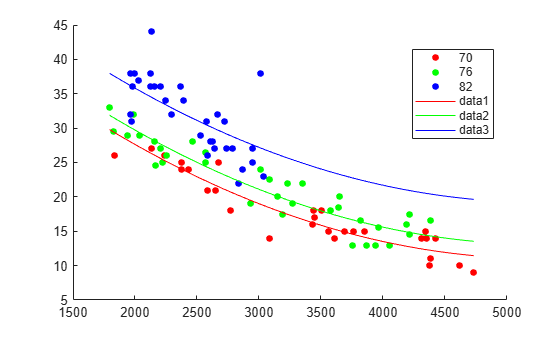Documentation

### This is machine translation

Mouseover text to see original. Click the button below to return to the English version of the page.

Note: This page has been translated by MathWorks. Click here to see
To view all translated materials including this page, select Country from the country navigator on the bottom of this page.

# feval

Predict responses of linear regression model using one input for each predictor

## Syntax

``ypred = feval(mdl,Xnew1,Xnew2,...,Xnewn)``

## Description

example

````ypred = feval(mdl,Xnew1,Xnew2,...,Xnewn)` returns the predicted response of `mdl` to the new input predictors `[Xnew1,Xnew2,...,Xnewn]`.```

## Examples

collapse all

Fit a mileage model to the `carsmall` data set, including the `Year` categorical predictor. Superimpose fitted curves on a scatter plot of the data.

Load the data set and fit the model.

```load carsmall tbl = table(MPG,Weight); tbl.Year = categorical(Model_Year); mdl = fitlm(tbl,'MPG ~ Year + Weight^2');```

Create a scatter plot of `MPG` versus `Weight`, grouped by `Year`.

`gscatter(tbl.Weight,tbl.MPG,tbl.Year);`Plot curves of the model predictions for the various years and weights by using `feval`.

```w = linspace(min(tbl.Weight),max(tbl.Weight))'; line(w,feval(mdl,w,'70'),'Color','r') line(w,feval(mdl,w,'76'),'Color','g') line(w,feval(mdl,w,'82'),'Color','b')```## Input Arguments

collapse all

Linear regression model object, specified as a `LinearModel` object created by using `fitlm` or `stepwiselm`, or a `CompactLinearModel` object created by using `compact`.

New predictor values, specified as a vector, matrix, table, or dataset array.

• If you pass multiple inputs `Xnew1,Xnew2,...,Xnewn` and each includes observations for one predictor variable, then each input must be a vector. Each vector must have the same size. If you specify a predictor variable as a scalar, then `feval` expands the scalar argument into a constant vector of the same size as the other arguments.

• If you pass a single input `Xnew1`, then `Xnew1` must be a table, dataset array, or matrix.

• If `Xnew1` is a table or dataset array, it must contain predictors that have the same predictor names as in the `PredictorNames` property of `mdl`.

• If `Xnew1` is a matrix, it must have the same number of variables (columns) in the same order as the predictor input used to create `mdl`. Note that `Xnew1` must also contain any predictor variables that are not used as predictors in the fitted model. Also, all variables used in creating `mdl` must be numeric or logical. To treat numerical predictors as categorical, identify the predictors using the `'CategoricalVars'` name-value pair argument when you create `mdl`.

Data Types: `single` | `double` | `table`

## Output Arguments

collapse all

Predicted mean values at `Xnew1,Xnew2,...,Xnewn`, returned as a numeric vector.

## Tips

• A `LinearModel` object is, mathematically, a function that estimates the relationship between the response and predictors. The `feval` function enables an object to behave like a function in MATLAB®. You can pass `feval` to another function that accepts a function input, such as `fminsearch` and `integral`. Also, `feval` can be simpler to use with a model created from a table or dataset array. When you have new predictor data, you can pass it to `feval` without creating a table or matrix.

## Alternative Functionality

• `predict` gives the same predictions as `feval` by using a single input argument with one observation in each row, rather than multiple input arguments with one input for each predictor variable. `predict` also gives confidence intervals on its predictions.

• `random` predicts responses with added noise.